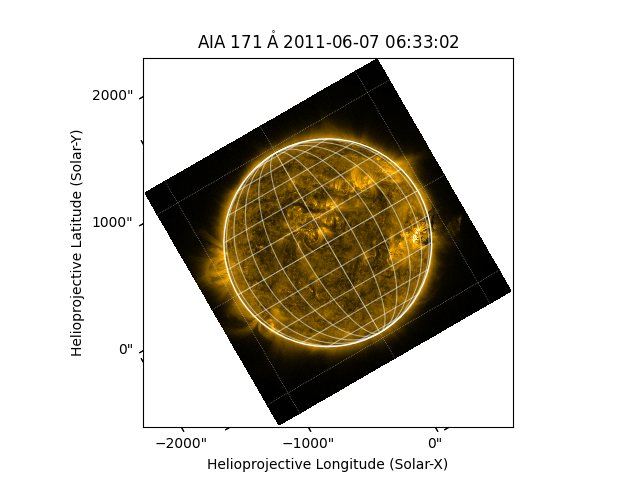# Rotating a Map¶

How to rotate a map.

import matplotlib.pyplot as plt

import astropy.units as u

import sunpy.data.sample
import sunpy.map


aia_map = sunpy.map.Map(sunpy.data.sample.AIA_171_IMAGE)


GenericMap provides the rotate() method which accepts an angle. This returns a rotated map and does not modify the original map. The data array size is expanded so that none of the original data is lost due to cropping.

aia_rotated = aia_map.rotate(angle=30 * u.deg)


Let’s now plot the results.

plt.figure()
aia_rotated.plot(clip_interval=(1, 99.99)*u.percent)
aia_rotated.draw_limb()
aia_rotated.draw_grid()
plt.show()Total running time of the script: ( 0 minutes 2.494 seconds)

Gallery generated by Sphinx-Gallery